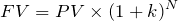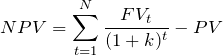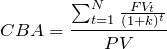# Project Selection Methods Top 5 Criteria

Project selection methods have a vital role during project phase. In fact during the project selection process a project manager rarely has any involvement. However, knowing the essentials of various project selection criteria adds value to the overall project execution. Moreover, those aspiring for the project management professional certification examination need to know the basics of project selection methodologies. Hence this post serves as an easy reference guide to understand the various project selection methods available.

## Project Selection Methods In PMBOK

Certainly, certification examination has few questions on project selection process. As a matter of fact the earlier editions of PMBOK guide did not have any reference to project selection methods.

Therefore, PMP aspirants had to rely on many online resources and other PMP exam preparatory reference books. Moreover, the PMBOK guide still has no mention of different project selection methods. But, The Guide to The Project Management Body of Knowledge 6th edition does mention financial measures as factors.

Accordingly, the PMBOK guide has listed five different financial tools as a measure for project success. The identified financial measures are not only a measure for but are also tools for project selection.

## Project Selection Methods Essential Concepts

In order to have good understanding of  project selection process, let us first know some basic terms. Accordingly, following paragraphs briefly define key financial concepts such as time value of money, present and future value.

Also, undermentioned paragraphs enumerate difference between terms like compounding and discounting.

### Time Value of Money

Time value of money is an equally important finance concept that helps understand economic or profitability project selection models.  It simply means that money received now has more value than the same amount received in future.

But, then what affects the value of money?

It is inflation and risk that significantly affect the value of future cash flow. Hence, any investor seeks compensation for the above factors that erode its returns.

Thus, risk free rate of interest compensates for time as well as inflation. Whereas risk premium provides a cover for investments risks.

Therefore, interest rate helps to convert future cash flow to an equivalent amount of money in the present time.

### Present Value

Present Value (PV) is the current value of future cash flows. Computing present value uses the principle of discounting.

Further, discounting is calculating the present value of future cash flows using a specified compound interest rate.

### Future Value

Future value (FV) is the value of current investment at any specified future date. Computing future value uses the principle of compounding.

Likewise, compounding is calculating the future value of current investment using specified compound interest.

### Present Value & Future Value Relationship

Following mathematical formula establishes the relationship between present value and future value.

Mathematical expression of Future Value (FV) is ;Similarly, Mathematical expression of Present Value (PV) is;Where; k = discounting factor / interest rate and N = Number of time periods

## Project Selection Methods Classification

Following is the list of various project selection methods.

1. Benefits Measurement Methods
1. Scoring Methods
1. Unweighted 0-1 Factor Model
2. Unweighted Factor Scoring Model
3. Weighted Factor Scoring Model
2. Economic Methods
1. Discounted Cash Flow (DCF) Methods
1. Net Present Value (NPV)
2. Internal Rate of Return (IRR)
3. Cost Benefit Analysis (CBA) / Profitability Index (PI)
2. Non-Discounted Cash Flow (Non-DCF) Methods
1. Payback Period (PB)
2. Discounted Payback Period
3. Return on Investment (ROI)
3. Murder Board
4. Peer Review
2. Constrained Optimized Methods
1. Linear Programming
2. Integer Programming
3. Dynamic Programming
4. Multi-Objective Programming

As mentioned above, the project selection methods list is quite exhaustive. However, from pmp exam point of view it is not mandatory to know all the calculation steps of above methods. Moreover, pmp exam tests for the application aspects of only the few tools mentioned above.

## Project selection Methods Top 5 Criteria

In fact, the most widely used project selection criteria is the economic model. Also, the other name for economic model for project selection is profitability model. Hence, in this post we will discuss only the following most important and widely used project selection methods.

1. Economic Methods
1. Discounted Cash Flow (DCF) Methods
1. Net Present Value (NPV)
2. Internal Rate of Return (IRR)
3. Cost Benefit Analysis (CBA) / Profitability Index (PI)
2. Non-Discounted Cash Flow (Non-DCF) Methods
1. Payback Period (PB)
2. Return on Investment (ROI)

## Net Present Value (NPV)

Certainly, the most widely used project selection method is Net Present Value (NPV). NPV is a profitability model that also uses discounted cash flow. In order to select a project it converts of future cash flows to their equivalent present value. NPV further compares the sum of equivalent present value of cash inflows to present cash outflow for decision-making.

### Net Present Value Formula

Following paragraphs enumerates the mathematical equation of net present value (npv).

NPV = [Sum of present value of all future cash inflows] – [Cash outflow]Where; t / N = time period and k = discounting factor

### Net Present Value Project Selection Criteria

A positive NPV means that the sum of present value of future cash flow is greater than the cash outflow. This implies that the project is profitable and hence acceptable.

if NPV is positive (i.e.greater than 0) Accept the project

A negative NPV means that the sum of present value of future cash flow is less than the cash outflow. This implies that the project is not-profitable and hence rejected.

if NPVis negative (i.e less than 0) Reject the project

When comparing NPV values of two or more projects always select a project with greater NPV. While comparing different NPV values a high NPV value indicates higher profitability.

Always select the project with greater NPV

Further, NPV equals to zero implies that the project generates cash inflow equal to the cash outflow. Hence it suggests that the project will neither be profitable nor it will be at loss.

## Internal Rate of Return (IRR)

The second most important project selection method is Internal Rate of Return (IRR). This project selection method also uses discounted cash flow . Moreover, mathematical formula of IRR is similar to that of NPV.

However, IRR is different from NPV; it is that interest rate at which NPV is equal to zero. The output of IRR is an interest rate at which the project neither makes profit nor any loss.

Thus in order to assess project profitability compare Internal Rate of Return (IRR) against the required interest rate (k).

### Internal Rate of Return Formula

IRR = [Sum of present value of all future cash inflows] – [Cash outflow] = 0

### Internal Rate of Return Project Selection Criteria

• Accept the project when IRR is greater than k
• Reject the project when IRR is less than k

## Cost Benefit Analysis (CBA)

Cost Benefit Analysis (CBA) is yet another popular project selection technique. Further, alternate names of cost benefit are Profitability Index (PI) and Benefit Cost Ratio (BCR) .

Moreover, it is a discounted cash flow project selection method that identifies time value of money.

Mathematically CBA is the ratio of present value of cash inflows to cash outflow .

### Cost Benefit Analysis Formula

Following is the mathematical expression of Cost Benefit Analysis (CBA)

CBA = Sum of Present Value of future cash Inflow / Project Cash outflow### Cost Benefit Analysis Project Selection Criteria

• Accept the project when CBA is greater than 1
• Reject the project when CBA is less than 1

## Return on Investment (ROI)

Next most important project selection criteria is Return on Investment (ROI). It is a financial ratio that uses net profit from an investment as a measure for project selection.

Even though ROI is a measure of profitability it does not use discounted cash flows.

In order to measure profitability of an investment proposal ROI uses accounting information in financial statements.

Return on investment is the ratio of average profit after tax to average investments.

However, various literature refer ROI formula in different ways. For example investopedia.com interprets ROI as ratio of profit from investments to cost of investment.

### Return on Investment Formula

IM Pandey in his book  Financial Management, Ninth Edition, describes the following formula for return on investment.

ROI = Average Income / Average Investment

Where; average income = average profit after tax; and average investment = average book value of the investment at the beginning and end of the time period.

### Return on Investment Project Selection Criteria

As a matter of fact, organizations establish a minimum rate of ROI as a mandatory criteria for project evaluation.

• Accept the project if return on investment is greater than the minimum ROI.
• Reject the project if it is less than the minimum ROI.

## Payback Period (PB)

Yet another most widely recognized project selection method is Payback period (PB) . PB indicates the number of years required to recover the initial project investment.

However, payback period does not take into account the effect of time value of money. Moreover, it is also does not discount the future cash flows.

Discounted payback period is one such variation of the above method. This process, discounts the future cash inflows but only until the project achieves its payback period. Moreover, it does not discount the complete series of cash inflows. Hence discounted payback is not a DCF based project selection method in its true sense.

### Payback Period Formula

Following is the mathematically expression of payback period;

Payback Period (PB) = Initial Investment / Annual Cash Inflow

The payback period formula mentioned above is valid if the project generates constant annual cash inflow. However, in order to arrive at the payback period for unequal cash inflow each year; add the cash inflows until the total is equal to the initial cash outflow.

### Payback Period Project Selection Criteria

As a management directive many organizations have a standard pre-determined payback period. During the project selection process compare payback period of a project against the standard payback period.

Accept a project if the payback is less than the standard payback period mandate. However, reject a project if the payback is greater than the standard mandate.

While selecting two projects always select a project with a lesser payback period.

## Project Selection Methods Summary Table

Following table is a summary of top 5 project management selection criteria

Project Selection MethodProject Selection CriteriaProject Selection Decision
Net Present Value (NPV)if NPV is greater than zeroAccept
if NPV is less than zeroReject
Internal Rate of Return (IRR)Greater than thresholdAccept
Less than thresholdReject
Cost Benefit Analysis (CBA)if CBA is greater than oneAccept
if CBA is less than oneReject
Return on Investment (ROI)Greater than thresholdAccept
Less than thresholdReject
Payback periodLess than thresholdAccept
Greater than thresholdReject

## Project Selection Methods Conclusion

Project selection methods as topic requires further study and deliberation. PMBOK 6th edition also lists project selection method like cost benefit as a data analysis tool. In fact, project management professional examination demands basic understanding of the subject. So far PMP exam has not asked for detailed calculation of any of the project selection methods. In fact application of any concept improves only when there is a good understanding of fundamentals.

## 4 thoughts on “Project Selection Methods Top 5 Criteria”

1.Gobinda Burman says:

Very Informative article…

1.Atul Gaur PMP says:

Thank You!

This site uses Akismet to reduce spam. Learn how your comment data is processed.